• 多普勒雷达的速度分辨率
2022-03-15 22:47:29

## 关于雷达距离分辨率与FFT点数的关系

雷达距离分辨率指的是最小能分辨的距离
比如最小分辨率是1m，那1.5m的目标在距离谱上就没法显示出来
而FFT点数是频谱的点数，即使FFT点数再多，也不可能使1.5m的目标在距离谱上表现出来，只能让距离为1m的目标频谱曲线更加的平滑

更多相关内容
• 对脉冲多普勒雷达的功能进行仿真，多目标，脉压，分辨率
• 目录距离多普勒(Range-Dopple Matrix)处理方法快时间维度处理(Range-FFT)慢时间维度处理(Doppler-FFT)RDM中距离分辨率速度分辨率推导方法参考资料 距离多普勒(Range-Dopple Matrix)处理方法   众所周知，距离...

# 距离多普勒(Range-Dopple Matrix)处理方法

众所周知，距离多普勒处理方法(Range-Dopple Matrix，简称RDM)是FMCW雷达进行多目标信息提取的有效手段，通过对雷达发送的多个周期的Chirp序列以及回波信息进行快时间维度和慢时间维度的处理，即可得到距离多普勒热力图，进而可以提取多目标的距离和速度信息。

插图来源于参考资料在FMCW的差拍信号中，我们知道，差拍信号的频率为
f m o v i n g B e a t = f s t a t i c B e a t ± f d = 2 f c R C t c ± 2 f v C (1) f_{movingBeat} = f_{staticBeat} \pm f_d = \frac{2f_cR}{Ct_c} \pm \frac{2fv}{C} \tag 1   其中 f m o v i n g B e a t f_{movingBeat} f s t a t i c B e a t f_{staticBeat} 分别为目标运动和静止状态下差拍信号的频率， f d f_d 为多普勒频率， f c f_c 为扫频带宽， R R 为目标距离， C C 为光速， t c t_c 为扫频周期， f f 为Chirp信号中心频率， v v 为目标速度。

## 快时间维度处理(Range-FFT)

快时间维度即单个周期的Chirp序列扫频周期时间很短，短到几乎可以将多普勒频率带来的影响忽略不计( t c t_c ↓，公式(1)中 f s t a t i c B e a t f_{staticBeat} 项占了主要的位置)，认为此时通过RDM热力图提取到的动目标在距离维度上的动目标差频 f m o v i n g B e a t f_{movingBeat} 与静目标差频 f s t a t i c B e a t f_{staticBeat} 近似相等，即 f m o v i n g B e a t ≈ f s t a t i c B e a t = 2 f c R C t c (2) f_{movingBeat} \approx f_{staticBeat} = \frac{2f_cR}{Ct_c} \tag 2   那么通过快时间维度的每一帧数据，提取频谱峰值对应的横坐标频率，即可对目标的距离进行求解；即 R = C t c 2 f c ⋅ f s t a t i c B e a t (3) R = \frac{Ct_c}{2f_c}\cdot f_{staticBeat} \tag 3   快时间维处理示意图如下

插图来源于参考资料## 慢时间维度处理(Doppler-FFT)

因为我们知道，在快时间维的处理中，认为速度带来的影响忽略不计，通过对多个Chirp序列进行多帧数据的堆积，此时在第二个维度上(即慢时间维度上，多帧数据对应的同一距离单元上)速度带来的频率影响就不可忽略，此时慢时间维度上求得的频率即为多普勒频率，即 f d = 2 f v C (4) f_d = \frac{2fv}{C} \tag 4   所以有 v = f d C 2 f (5) v = \frac{f_dC}{2f} \tag 5
慢时间维处理示意图如下

插图来源于参考资料慢时间维度的处理是经过多个Chirp序列积累后对同一距离单元进行FFT的结果，故称为慢时间维度，

为什么是同一距离单元？
因为Range-FFT中同一个横坐标对应相同的 f m o v i n g B e a t f_{movingBeat} ，快时间维度下 f m o v i n g B e a t f_{movingBeat} 约等于 f s t a t i c B e a t f_{staticBeat} ，由公式(2)和公式(3)可知，对应同一距离单元

快时间维度和慢时间维度处理总览

插图来源于参考资料经过处理后可得到如下的距离多普勒热力图(Range-Dopple Heat Map)

插图来源于参考资料# RDM中距离分辨率和速度分辨率推导方法

网上关于RDM方法中距离分辨率和速度分辨率推导的资料实在太少，几乎都是两个长得不太好看公式直接糊你脸上，我的感受就是老人、地铁、看手机.jpg(此处省略表情包)，于是决定记录下推导过程，正所谓难者不会，会者不难。
首先回顾下数字信号处理中第K个采样点的频率 f k f_k 与采样频率 f s f_s 间的关系，我们知道，第K个采样点的角频率服从如下关系
ω k = k N s ⋅ 2 π = Ω ⋅ T s = 2 π f k ⋅ 1 f s \omega_k = \frac{k}{N_s}\cdot2\pi = \Omega\cdot T_s = 2 \pi f_k\cdot \frac{1}{f_s}
其中 N s N_s 为采样点数， Ω \Omega 为模拟角频率， T s T_s 为采样频率，我们取出等式中的第二项和第四项，有
k N s ⋅ 2 π = 2 π f k ⋅ 1 f s \frac{k}{N_s}\cdot2\pi =2 \pi f_k\cdot \frac{1}{f_s}   可得第K个采样点的频率 f k f_k 与采样频率 f s f_s 间的关系为
f k = k ⋅ f s N s (6) f_k = k\cdot \frac{f_s}{N_s} \tag 6
到此就可以正式展开距离分辨率和速度分辨率的推导方法了，上一部分我们说到快时间维度的Range-FFT和慢时间维度的Doppler-FFT，有两个结论性的公式
f m o v i n g B e a t ≈ f s t a t i c B e a t = 2 f c R C t c (7) f_{movingBeat} \approx f_{staticBeat} = \frac{2f_cR}{Ct_c} \tag 7 f d = 2 f v C (8) f_d = \frac{2fv}{C} \tag 8   假设上一部分中距离多普勒热力图中， n 1 n_1 为Range-FFT(快时间维度)中目标对应的坐标序列号， n 2 n_2 为Doppler-FFT(慢时间维度)中同一目标对应的坐标序列号，则依照公式(6)可得
f m o v i n g B e a t = n 1 N s ⋅ f s (9) f_{movingBeat} = \frac{n_1}{N_s} \cdot f_s \tag 9 f d = n 2 N C h i r p ⋅ 1 t c (10) f_d = \frac{n_2}{N_{Chirp}} \cdot \frac{1}{t_c} \tag {10}   其中 N C h i r p N_{Chirp} 为慢时间维度处理中Chirp序列的积累个数；公式(9)类比公式(6)，比较好理解，公式(10)也是类比公式(6)，只不过此时在慢时间维度上采样总数是积累的Chirp序列的总数，采样频率是每一个Chirp序列扫频周期的倒数，即 1 t c \frac{1}{t_c}
由此以来，分别联立公式(7)和公式(9)，联立公式(8)和公式(10)，可得
2 f c R C t c = n 1 N s ⋅ f s \frac{2f_cR}{Ct_c} = \frac{n_1}{N_s} \cdot f_s 2 f v C = n 2 N C h i r p ⋅ 1 t c \frac{2fv}{C} = \frac{n_2}{N_{Chirp}} \cdot \frac{1}{t_c}   可解得
R = C 2 f C ⋅ t c ⋅ n 1 N s ⋅ f s (11) R = \frac{C}{2f_C}\cdot t_c\cdot \frac{n_1}{N_s}\cdot f_s \tag{11}
v = C 2 f ⋅ n 2 N C h i r p ⋅ 1 t c (12) v = \frac{C}{2f} \cdot \frac{n_2}{N_{Chirp}}\cdot \frac{1}{t_c}\tag{12}   因为
t c = N s ⋅ T s = N s f s (13) t_c = N_s\cdot T_s = \frac{N_s}{f_s} \tag{13} t s e q = N C h i r p ⋅ t c (14) t_{seq} = N_{Chirp}\cdot t_c\tag{14}   将(13)代入(11)，将(14)代入(12)，可得
R = C 2 f c ⋅ n 1 R = \frac{C}{2f_c} \cdot n_1 v = C 2 f t s e q ⋅ n 2 v = \frac{C}{2ft_{seq}} \cdot n_2   此时，就得到了距离分辨率和速度分辨率，分别为
R r e s = C 2 f c R_{res} = \frac{C}{2f_c} v r e s = C 2 f N C h i r p t c = C 2 f t s e q v_{res} = \frac{C}{2fN_{Chirp}t_c} = \frac{C}{2ft_{seq}}

# 仿真程序代码

仿真程序代码如下，作者：Elias.J

%%%%%%%%%%%%%%%%%%%%%%%%%%%%%%%%%%%%%%%%%%%%%%%%%%%%%%%%%%%%%%%%%%%%%
% File name: RDM.m
% Author: Elias.J@CSDN
% CSDN: https://blog.csdn.net/qq_41248471
%%%%%%%%%%%%%%%%%%%%%%%%%%%%%%%%%%%%%%%%%%%%%%%%%%%%%%%%%%%%%%%%%%%%%
%% Initial operation
close all;
clc;
tarR = [15 25];    %target range
tarV = [-3 10];     %target velocity
c = 3*10^8;
f0 = 24.25*10^9;
T = 0.0002;   %chirp Sweep Time
B = 400*10^6;
L = 128;            %slow-time dimension,num of chirps
N = 128;           %fast-time dimension,num of samples
Npad = 1;          %padding in order to improve measure precision
Lpad = 1;         %padding in order to improve measure precision
%% generate receive signal
S1 = zeros(L,N);
for l = 1:L
for n = 1:N
S1(l,n) = 500*exp(1i*2*pi*((2*B*(tarR(1)+tarV(1)*T*l)/(c*T)+(2*f0*tarV(1))/c)*T/N*n+((2*f0)*(tarR(1)+tarV(1)*T*l))/c));
end
end
S1 = awgn(S1,20);

S2 = zeros(L,N);
for l = 1:L
for n = 1:N
S2(l,n) = 500*exp(1i*2*pi*((2*B*(tarR(2)+tarV(2)*T*l)/(c*T)+(2*f0*tarV(2))/c)*T/N*n+((2*f0)*(tarR(2)+tarV(2)*T*l))/c));
end
end
S2 = awgn(S2,20);
%% range fft processing
hanning1 = hanning(N,'periodic');
% hanning1 = ones(N,1);
sigRWin = zeros(L,N);
for ii = 1:L
end
for ii = 1:L
end
%% doppler fft processing
hanning2 = hanning(L,'periodic');
% hanning2 = ones(N,1);
for ii = 1:N*Npad
sigDWin(:,ii) = hanning2.*sigRfft(:,ii);
end
for ii = 1:N*Npad
end
%% Visualization
xlabel('Range/m'),ylabel('Frame');
figure,mesh(abs(sigRfft)),title('Range-FFT');
xlabel('距离/m'),ylabel('速度/mps');
xlim([0 45]);
ylim([-18 18]);
xlabel('距离/m'),ylabel('速度/mps'),zlabel('幅值/dB');

%% Window Test

hanning1 = ones(N,1);
sigRWin = zeros(L,N);
for ii = 1:L
end
for ii = 1:L
end

hanning2 = ones(N,1);
for ii = 1:N*Npad
sigDWin(:,ii) = hanning2.*sigRfft(:,ii);
end
for ii = 1:N*Npad
end

figure(1),
xlabel('距离/m'),ylabel('速度/mps');
title('加矩形窗');
figure(2),
xlim([0 45]);
ylim([-18 18]);
xlabel('距离/m'),ylabel('速度/mps'),zlabel('幅值/dB');
title('加矩形窗');

hanning1 = hanning(N,'periodic');
sigRWin = zeros(L,N);
for ii = 1:L
end
for ii = 1:L
end

hanning2 = hanning(L,'periodic');
for ii = 1:N*Npad
sigDWin(:,ii) = hanning2.*sigRfft(:,ii);
end
for ii = 1:N*Npad
end

figure(1),
xlabel('距离/m'),ylabel('速度/mps');
title('加汉宁窗');
figure(2),
xlim([0 45]);
ylim([-18 18]);
xlabel('距离/m'),ylabel('速度/mps'),zlabel('幅值/dB');
title('加汉宁窗');

hanning1 = hamming(N,'periodic');
sigRWin = zeros(L,N);
for ii = 1:L
end
for ii = 1:L
end

hanning2 = hamming(L,'periodic');
for ii = 1:N*Npad
sigDWin(:,ii) = hanning2.*sigRfft(:,ii);
end
for ii = 1:N*Npad
end

figure(1),
xlabel('距离/m'),ylabel('速度/mps');
title('加汉明窗');
figure(2),
xlim([0 45]);
ylim([-18 18]);
xlabel('距离/m'),ylabel('速度/mps'),zlabel('幅值/dB');
title('加汉明窗');

hanning1 = blackman(N,'periodic');
sigRWin = zeros(L,N);
for ii = 1:L
end
for ii = 1:L
end

hanning2 = blackman(L,'periodic');
for ii = 1:N*Npad
sigDWin(:,ii) = hanning2.*sigRfft(:,ii);
end
for ii = 1:N*Npad
end

figure(1),
xlabel('距离/m'),ylabel('速度/mps');
title('加布莱克曼窗');
figure(2),
xlim([0 45]);
ylim([-18 18]);
xlabel('距离/m'),ylabel('速度/mps'),zlabel('幅值/dB');
title('加布莱克曼窗');
%% 2D CA-CFAR
Pfa = 10^(-6);
Rres_rdm = c/(2*B);
Vres_rdm = c/(2*24.25*10^9*T*L);
Range_Dim = Rres_rdm*[1:N];
Velocity_Dim = Vres_rdm*([1:L] - L/2);
Range_Dopple_Map = abs(sigDfft);

handleWindow_r = 9;
handleWindow_c = 9;
handleWindow = zeros(handleWindow_r,handleWindow_c);
proCell_r = 5;
proCell_c = 5;
proCell = zeros(proCell_r,proCell_c);
[r c] = size(Range_Dopple_Map);
CFAR_Map_r = r - (handleWindow_r-1);
CFAR_Map_c = c - (handleWindow_c-1);
CFAR_Map = zeros(CFAR_Map_r,CFAR_Map_c);
referCellNum = handleWindow_r*handleWindow_c - proCell_r*proCell_c;
alpha = referCellNum*(Pfa^(-1/referCellNum) - 1);
for i = 1:CFAR_Map_r
for j = 1:CFAR_Map_c
handleWindow = Range_Dopple_Map(i:i+handleWindow_r-1,j:j+handleWindow_c-1);
proCell = handleWindow(1+(handleWindow_r - proCell_r)/2:handleWindow_r - (handleWindow_r - proCell_r)/2,1+(handleWindow_c - proCell_c)/2:handleWindow_c - (handleWindow_c - proCell_c)/2);
Beta = (sum(sum(handleWindow)) - sum(sum(proCell)))/(referCellNum);
CFAR_Map(i,j) = alpha*Beta;
end
end

CFAR_MapRange_Dim = Range_Dim(1 + handleWindow_r - proCell_r:N - (handleWindow_r - proCell_r));
CFAR_MapVelocity_Dim = Velocity_Dim(1 + handleWindow_c - proCell_c:N - (handleWindow_c - proCell_c));
figure,subplot(121);
mesh(Range_Dim,Velocity_Dim,10*log10(Range_Dopple_Map));
xlabel('距离/m'),ylabel('速度/mps'),zlabel('幅值/dB');
title('2D-FFT')
subplot(122);
mesh(CFAR_MapRange_Dim,CFAR_MapVelocity_Dim,10*log10(CFAR_Map));
xlabel('距离/m'),ylabel('速度/mps'),zlabel('幅值/dB');
title('2D-CFAR检测判决门限')

figure,subplot(121);
image(Range_Dim,Velocity_Dim,10*log10(Range_Dopple_Map));
xlabel('距离/m'),ylabel('速度/mps');
title('距离多普勒图');
subplot(122);
image(CFAR_MapRange_Dim,CFAR_MapVelocity_Dim,10*log10(CFAR_Map));
xlabel('距离/m'),ylabel('速度/mps');
title('2D-CFAR检测判决门限')

CRange_Dopple_Map = Range_Dopple_Map(1 + handleWindow_r - proCell_r:N - (handleWindow_r - proCell_r),1 + handleWindow_c - proCell_c:N - (handleWindow_c - proCell_c));
[comR comC] = find(CRange_Dopple_Map < CFAR_Map);
for i = 1:length(comR)
CRange_Dopple_Map(comR(i),comC(i)) = 0;
end

figure,mesh(CFAR_MapRange_Dim,CFAR_MapVelocity_Dim,CRange_Dopple_Map);
xlabel('距离/m'),ylabel('速度/mps'),zlabel('幅值');
figure,image(CFAR_MapRange_Dim,CFAR_MapVelocity_Dim,CRange_Dopple_Map);
xlabel('距离/m'),ylabel('速度/mps');


# 参考资料

展开全文• 今天进入第11章-脉冲雷达的工作机理。看看脉冲体制的雷达是如何工作的。本章目录如下：11. 脉冲雷达的工作机理11.1脉冲发射的优点11.2脉冲波形11.2.1载波频率11.2.2脉冲宽度11.2.3脉内调制11.2.4脉冲重复频率11.3...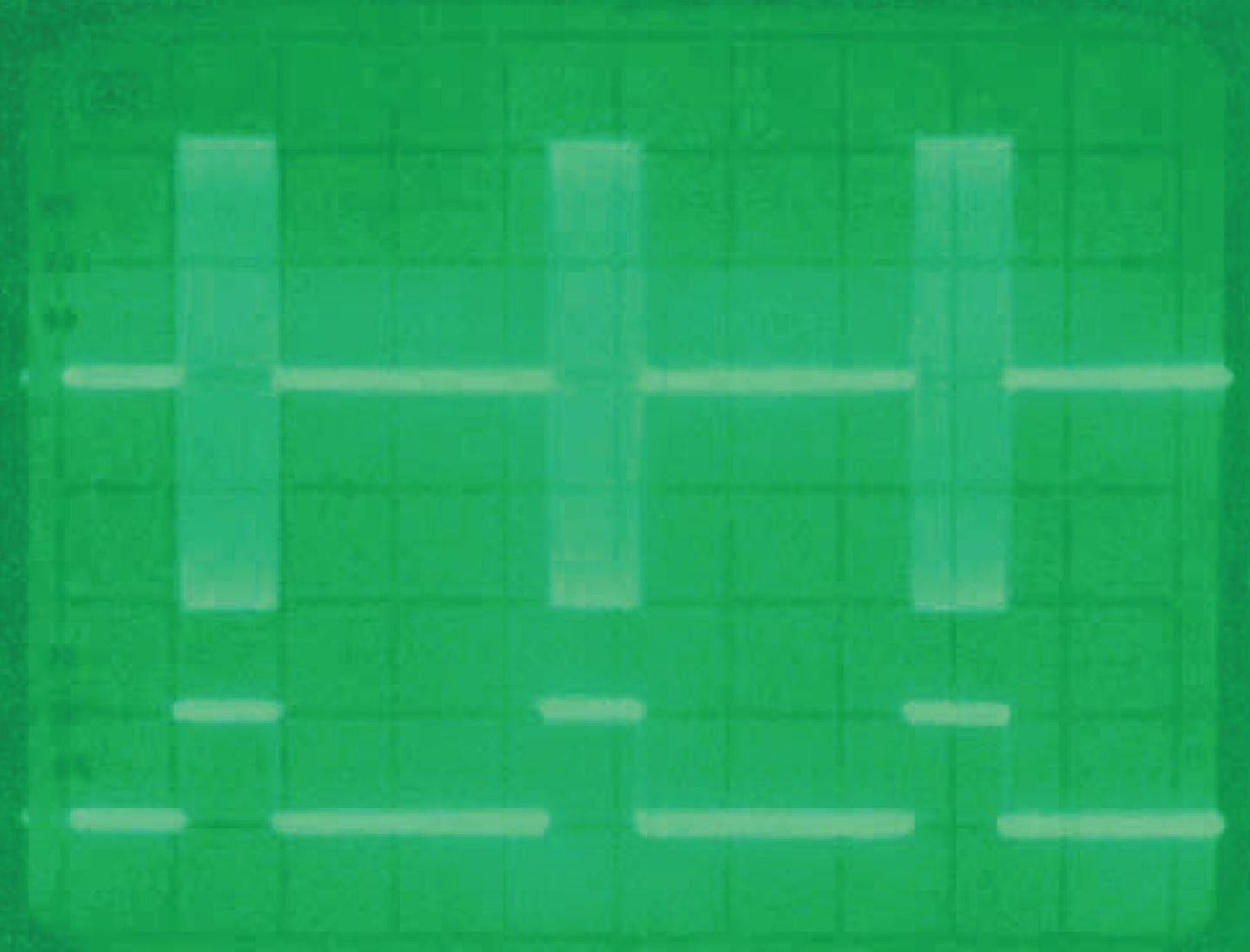今天进入第11章-脉冲雷达的工作机理。看看脉冲体制的雷达是如何工作的。本章目录如下：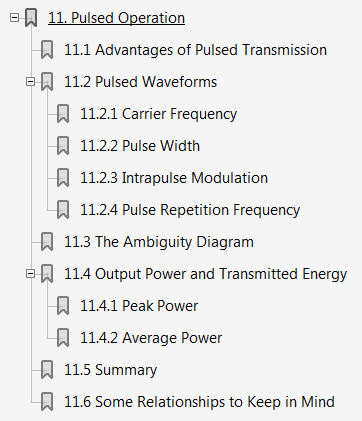11. 脉冲雷达的工作机理

11.1脉冲发射的优点

11.2脉冲波形

• 11.2.1载波频率
• 11.2.2脉冲宽度
• 11.2.3脉内调制
• 11.2.4脉冲重复频率

11.3模糊图

11.4输出功率和发射能量

• 11.4.1峰值功率
• 11.4.2平均功率

11.5总结

11.6要记住的一些关系式

说到脉冲雷达，我们首先说说为什么使用脉冲雷达，哪些应用场合使用脉冲(PW)雷达，和它对立的是连续波(CW)雷达，我们来对比一下脉冲雷达和连续波雷达的优缺点。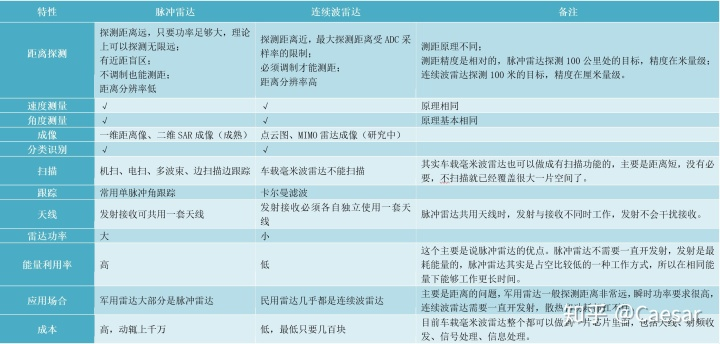脉冲雷达，顾名思义就是发射脉冲波的雷达。脉冲雷达发射短而有力的脉冲，并在静默期间接收回波信号。见下图，发射波的峰值很高，接收波的峰值很低，往往淹没在噪声中。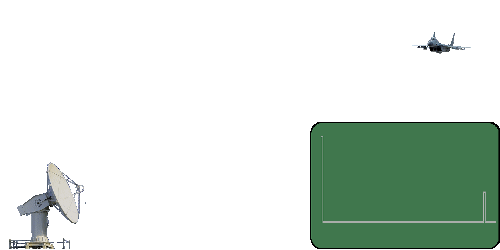大家注意看图中的右下角。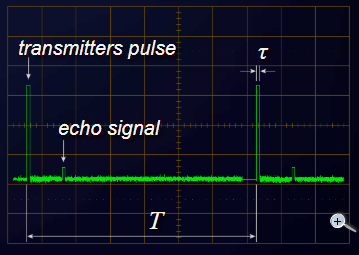图中的T就是脉冲重复周期PRT，其倒数就是脉冲重复频率。PRT一般是毫秒量级。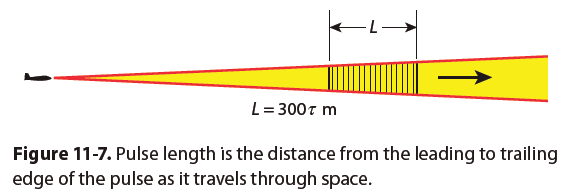还有一个概念是脉冲宽度，顾名思义，就是每个脉冲的宽度。既可用时间表示，也可以用长度表示。如图11-7，用时间表示为τ，一般是微秒量级，单位取微秒，乘以光速就是距离L = 300τ(m)。

PRF的选择至关重要，因为它决定了雷达观测到的距离和多普勒频率是否模糊，以及在何种程度上模糊。距离模糊产生的原因如下。雷达无法直接判断某一特定回波属于哪个发射脉冲。如果PRI相对于目标距离足够长，一个脉冲的所有回波都能在发送下一个脉冲之前被接收到，但是如果PRI比目标距离短，一个回波就可能属于任何一个之前的脉冲数。因此，雷达观测到的距离可能是模糊的。如下图所示。速度模糊的原因也类似。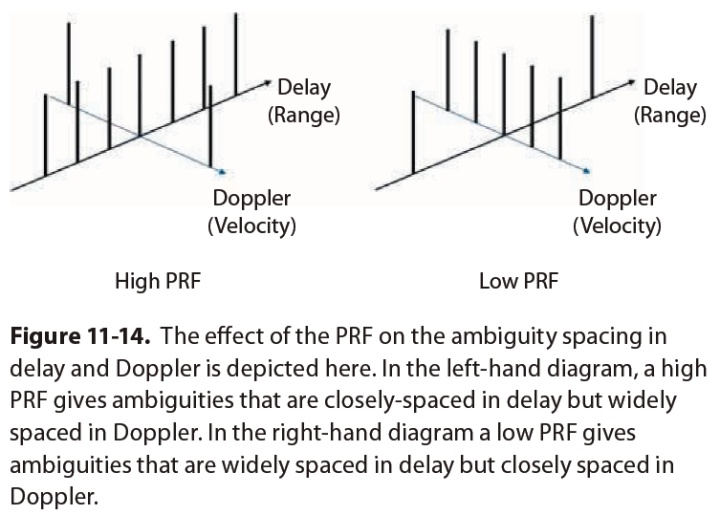看不懂也没关系，关于模糊的问题在第六大篇会单独讲解，这里先把概念引出。

还有调制的问题，分为脉内调制和脉间调制。脉内调制大部分采用线性调频FM。脉冲雷达调制是为了增加分辨力，连续被调制是为了能测距，原理后面的章节很快会介绍到。

最后还有就是峰值功率和平均功率的关系，很简单的，看下图就行。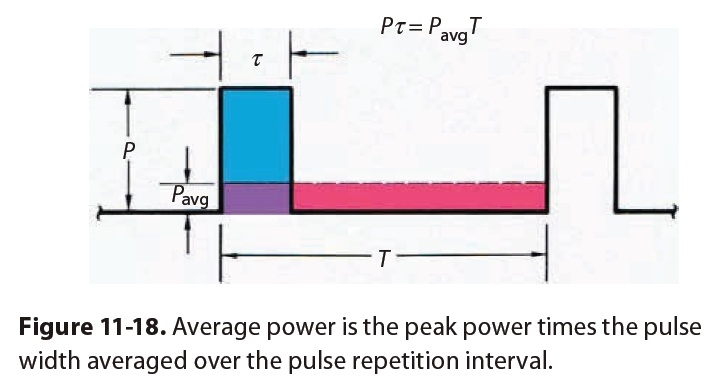=======================================================

好了，本章的扩展阅读和问题如下：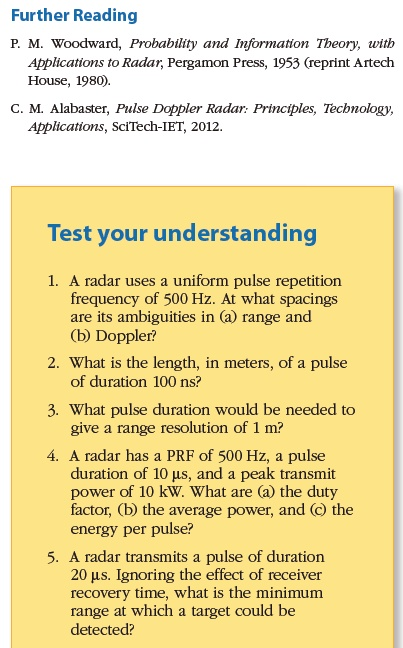1. 雷达重频为500Hz。它的(a)最大不模糊距离和(b)最大不模糊多普勒频率是多少？

答：(a) c*(1/500Hz)/2=300km，除以2是因为雷达波是往返的

(b) 500Hz/2=250Hz，除以2是因为奈奎斯特采样定理

2. 持续时间为100ns的脉冲的长度是多少?

答：这个就是电磁波在100ns内走的距离。光速乘时间可计算得30m。

3.需要多少脉冲持续时间才能获得1米的距离分辨率?

答：没有使用脉冲压缩的情况下，2*1m/c=6.67ns

4. 雷达的PRF为500Hz，脉冲持续时间为10us，峰值传输功率为10kW。(a)占空比(b)平均功率(c)单个脉冲的能量是多少?

答： (a)10e-6 * 500 = 0.5%

(b)10000W*0.5% = 50W

(c)10kW*10us=0.1J

5. 雷达发出持续时间为20 us的脉冲。忽略接收机恢复时间的影响，目标可被检测到的最小距离是多少?

答：没有使用脉冲压缩的情况下，最小距离为c*t/2=3000m。

展开全文• 基于原子范数最小化的亚奈奎斯特雷达分辨率延迟多普勒估计
• 针对连续波多普勒雷达外弹道测速的特点，为了解决频率分辨率与采样频率影响多普勒雷达速度测量精度的问题，提出应用快速傅里叶变换的频谱分析法。该方法基于目标弹道升、降规律对估计频率进行线性调整，能有效提高...
• 多基站雷达距离和多普勒分辨率；距离模糊函数；多普勒模糊函数 Range and Doppler resolution of multi base station radar; Distance ambiguity function; Doppler ambiguity function
• 基于多普勒雷达传感器，以STM32单片机为主控芯片，根据不同模块检测距离的不同，使用不同多普勒雷达传感器实现对远近距离车辆行驶速度及方向的测量。 1、基础知识 无线电探测和测距，是用无线电(电磁波)方法发现目标...
基于多普勒雷达传感器，以STM32单片机为主控芯片，根据不同模块检测距离的不同，使用不同多普勒雷达传感器实现对远近距离车辆行驶速度及方向的测量。


## 1、基础知识

#### 雷达

对于雷达的详细介绍请点这里

#### 多普勒理论

波是由频率和振幅所构成，而无线电波是随着物体而移动的，当无线电波在行进的过程中，碰到物体时，该无线电波会被反弹，而且其反弹回来的波，其频率及振幅都会随着所碰到的物体的移动状态而改变。若无线电波所碰到的物体时固定不动的，那么所反弹回来的无线电波其频率是不会改变的。然而，若物体朝着无线电线发射的方向前进时，此时所反弹回来的无线电波会被压缩，因此该电波的频率会随之增加；反之，若物体是朝着远离无线电波方向行进时，则反弹回来的无线电波，其频率则会随之减小。

## 2、傅里叶变换

我们在绝大多数傅里叶变换的应用都是采用离散傅里叶变换（DFT），更确切的说，是它的快速算法FFT。下面简要介绍一下这些傅里叶变换是什么，有什么联系。

#### 2.1 傅里叶变换（Fourier transform）

一种线性的积分变换，可以理解为一种从时间到频率的变化或其相互转化。傅里叶分析方法是信号分析的最基本方法，傅里叶变换是傅里叶分析的核心，通过它把信号从时间域变换到频率域，进而研究信号的频谱结构和变化规律。

关于傅里叶变换的具体讲解可以看下面这篇文章，我觉得讲的还是挺详细的：深入浅出的讲解傅里叶变换(真正的通俗易懂)

那有了傅里叶变换了，为什么还要搞出个离散傅里叶变换呢？
不管是傅里叶变换还是逆变换都是正负无穷的连续积分，这在数学公式上尚可以根据微分及极限求解公式进行计算，但却不太方便在计算机上实现，因为计算机是一个离散系统，那离散傅里叶变换就是用来解决这个问题的，使用计算机这个离散系统进行有限近似计算。

#### 2.2 离散傅里叶变换（Discrete Fourier Transform，DFT）

离散傅里叶变换，是傅里叶变换在时域和频域上都呈现离散的形式，将时域信号的采样变换为在离散时间傅里叶变换（DTFT）频域的采样。

离散时间傅里叶变换(Discrete Time Fourier Transform，DTFT
指无限长离散信号如何进行傅里叶变换。

离散时间傅里叶变换中原始信号是无限长的，即使采样后，采样点也是无限个，可以认为周期为无限长，因此它的频谱就趋向于连续，而连续的频谱同样不利于计算机处理，所以频率也要离散化才行，所需要的技术就是离散傅里叶变换DFT，即具有周期特性离散信号的傅里叶级数(就是将无限长的离散信号进行截短至N个采样点，然后将这个N个采样点进行周期延拓，变成周期信号，这样其频率就离散了。

在实际应用中通常采用快速傅里叶变换以高效计算DFT。

#### 2.3 快速傅里叶变换 (fast Fourier transform，FFT),

即利用计算机计算离散傅里叶变换（DFT)的高效、快速计算方法的统称。采用这种算法能使计算机计算离散傅里叶变换所需要的乘法次数大为减少，特别是被变换的抽样点数N越多，FFT算法计算量的节省就越显著。

有关DFT和FFT更详细的讲解可以看下面这篇文章：
全面解析傅立叶变换（非常详细）

## 4、雷达传感器

接下来我们介绍两款常用的雷达传感器K-LC6和K-MC1。用于测量物体存在、速度、运动方向、距离信息。

#### 4.1 K-LC6

24GHz雷达传感器，窄波束角度K波段带VCO雷达传感器。一种双通道多普勒雷达模块，具有不对称窄波束，用于中短距离传感器。非常适合人和车辆的移动和存在传感器。
探测距离：人>24米；车>62米。（近距离

●该模块包括一个射频低噪声放大器（LNA），以获得最佳的信噪比性能。双中频I和Q允许运动方向检测和高性能信号处理。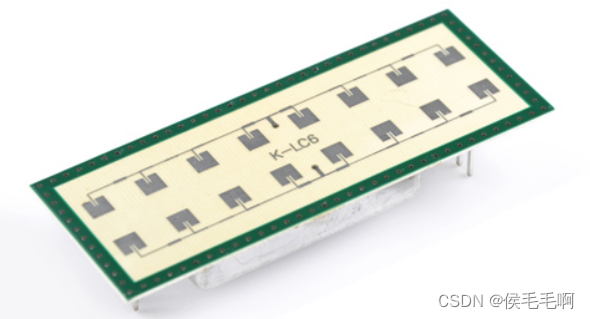#### 4.2 K-MC1

24GHz雷达传感器，带VCO窄波束K波段雷达传感器，60贴片多普勒模块，具有非对称窄波束，用于远程传感器。
探测距离：人56米；车>150米。（远距离

●模块包括一个射频低噪声放大器和两个47dB中频前置放大器，用于I和Q通道。此功能将显著减少对外部模拟电子设备的需求。对于特殊信号条件应用，提供额外的缓冲混频器直流输出。这大大提高了FSK测距应用的灵活性。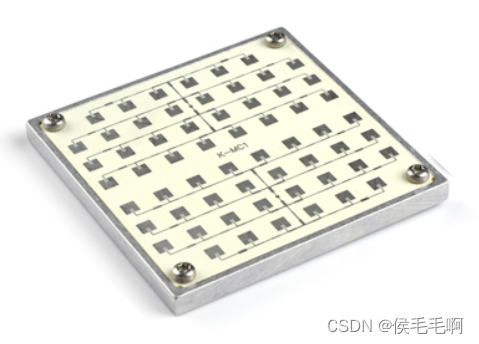展开全文• 针对由运动目标在步进频间不同频点引入的多普勒频率跨速度分辨单元走动问题，提出了基于Keystone变换的补偿方法，即通过时域的伸缩变换来补偿多普勒频率走动问题，并研究了基于多通道Keystone变换和基于装订信息的两...
• 第五章 脉冲多普勒雷达 5.4 脉冲多普勒雷达的信号处理 5.4.1 概述 5.4.2 抑制各种杂波的滤波器和恒虚警处理 　脉冲多普勒雷达接收机是一个复杂的信号处理系统，在这一系统中包括对发射机泄漏和高度杂波的抑制，...
• ## 【雷达信号处理】---雷达分辨率

千次阅读 多人点赞 2021-06-19 17:23:26
关键词：时延分辨率,距离分辨率,时延分辨常数TRC,频谱持续宽度FSP,距离分辨常数,多普勒分辨率,速度分辨率,频率分辨常数FRC,时间持续宽度(有效相关时间)TSP,速度分辨常数 距离分辨率 假定两个目标在同一角度保持...
• 测量目标距离仍然是大多数雷达系统的基本用途。然而，雷达系统在其组成方式、使用的信号、可捕获的信息以及如何在不同的应用中使用这些信息等方面都有了显著的发展。雷达广泛应用于军事和民用领域，包括：监视(威胁...
• 这篇文章的前提条件是在大家已经清楚整个雷达仿真的基本原理并且能自己熟练的根据公式写出雷达信号仿真的距离和相位变化信息。如果这里概念不清楚，请看这qwe14789cn：雷达信号仿真的基本原理——时延与相位的变化​...
• 本文运用多普勒雷达产品和石家庄加密自动站雨量资料对2004年、2005年汛期发生在河北省境内的三场暴雨天气进行了分析，结果发现：利用多普勒雷达产品时空分辨率高、产品丰富和速度场变化较降水变化超前的优点，配合...
• 基本原理与综述1.1 MTI雷达和PD雷达的应用1.2 多普勒效应例1 利用多次多普勒测量信息确定目标运动轨迹1.3 多普勒处理基础1.3.1 分辨率的考虑1.3.2 面杂波环境中的MTI多普勒滤波器1.3.3 面杂波和体杂波环境中的MTI...
• 毫米波雷达学习（一） 毫米波雷达学习（二） 毫米波雷达学习（三）我们在这提一下离散傅里叶变换，假设有一个离散信号，这个向量的每个样本以w角频率旋转，这样每个样本相同时间都旋转了同样角度。这时我们使用...
• 对于机载侧视雷达，若是实孔径雷达，其分辨率将受到很大的...当时，有些科学家想突破经典分辨率的限制，提出了一些新的设想：利用目标与雷达的相对运动所产生的多普勒频移现象来提高分辨力；用线阵天线概念证明运动...
• 基本原理与综述1．1 MTI雷达和PD雷达的应用1．2 多普勒效应例1 利用多次多普勒测量信息确定目标运动轨迹1．3 多普勒处理基础1．3．1 分辨率的考虑1．3．2 面杂波环境中的MTI多普勒滤波器1．3．3 面杂波和体杂波环境...
• 一、雷达信号流程建模 波形产生------>发射机------->射频天线======> (WaveForm Generator) (Transmitter) (Transmit Array) **== 信道 ==** 信号处理<---------接收机<----接收天线<====== ...
• 8.1.1 多普勒效应多普勒效应是奥地利物理学家J.Doppler 1842年首先从运动着的发声源中发现的现象，定义为"当接收者或接收器与能量源处于相对运动状态时，能量到达接收者（器）时频率的变化"。一个例子是：当一辆紧急...
• 利用相干激光雷达探测...在4 km光纤延迟时,本系统最低探测速度为0.5 mm/s,速度分辨率达到毫米每秒量级,频率分辨率达到千赫兹量级。利用微多普勒信息探测技术,实现了探测物体表面的微动状态信息和识别运动状态的目的。
• 在开始之前, 让我们先来看一下有关啁啾和雷达分辨率的三个主要测量维度. 啁啾 啁啾(Chirp)是指频率随时间而改变（增加或减少）的信号, 这一术语可以与扫频信号(Sweep signal)互换使用. 它通常用于声纳、雷达和激光....
• 对基于法布里珀罗(F-P)标准具四边缘技术的双频率多普勒测风激光雷达进行研究。简要分析了F-P标准具四边缘双频率风速测量原理。详细介绍了基于F-P标准具四边缘双频率多普勒激光雷达系统结构。对发射激光双频率间隔、...
• 测风激光雷达，尤其是相干多普勒激光雷达CDL属于主动大 气遥感，具有体积小，风场测量精度高，时间和空间分辨率 出色等特点。和传统的微波测风雷达相比，尤其在晴空风场 风速测量例如晴空湍流， CDL有丌可替代的...激光雷达
• 一、24GHz与77GHz频段比较 1、 24GHz频段 我们感兴趣的频段见图，24.0...在短程雷达中，24GHz频段的NB和UWB雷达已经应用于传统的汽车传感器上。通常NB雷达可以完成盲点检测等简单应用，但在大多数情况下包括超短距...
• 基于建立的车载直接探测激光雷达系统,对接收光学鉴频器进行了研究。针对边界层、对流层和平流层不同的气溶胶和大气分子浓度以及风速动态范围,同时采用直接探测的两种主要技术。利用多光束菲索(Fizeau)干涉仪(MFI)和...
• 六、实验思考题及心得 脉冲压缩提高了雷达分辨率，从信号幅度上分析，第一个目标由于距离比较近，雷达发射与接收是分开进行的，当回波到达是雷达还处于发射状态，雷达处于闭锁期不能接收回波，从而第一个目标的回波......